Courses

# Test: Thermochemistry

## 30 Questions MCQ Test Chemistry for JEE | Test: Thermochemistry

Description
This mock test of Test: Thermochemistry for Class 11 helps you for every Class 11 entrance exam. This contains 30 Multiple Choice Questions for Class 11 Test: Thermochemistry (mcq) to study with solutions a complete question bank. The solved questions answers in this Test: Thermochemistry quiz give you a good mix of easy questions and tough questions. Class 11 students definitely take this Test: Thermochemistry exercise for a better result in the exam. You can find other Test: Thermochemistry extra questions, long questions & short questions for Class 11 on EduRev as well by searching above.
QUESTION: 1

### For which of the following change ΔH ≠ ΔE ?

Solution:

∆H = ∆U+∆ngRT
For ∆H not equal ∆U, ∆ng should not equal zero.
This happens only in option d, where ∆ng  = -2

QUESTION: 2

### ΔrH of which of the following reactions is zero ?

Solution:

In equation d, H+(aq) is formed from H2(g). Enthalpy of formation of both entity is considered to be zero. So, ∆rH (∆Hproduct - ∆Hreactant) is zero.

QUESTION: 3

### How much heat will be required at constant pressure to form 1.28 kg of CaC2 from CaO(s) & C(s) ? Given : ΔfH°(CaO, s) = -152 kcal/mol ΔfH°(CaC2, s) = -14 kcal/mol ΔfH°(CO, g) = -26 kcal/mol

Solution:

Reaction is CaO + C → CaC2+CO
1.28 kg of CaC2 means 20 mole CaC2 is needed
For 1 mole dH=-26-14-(-152)=112
For 20 Mole 112×20=2240

QUESTION: 4

50.0 mL of 0.10 M HCl is mixed with 50.0 mL of 0.10 M NaOH. The solution temperature rises by 3.0°C Calculate the enthalpy of neutralization per mole of HCl. [take proper assumptions]

Solution:

No. of moles of HCl = 5 millimoles
No. of moles of NaOH = 5 millimoles
Mass of solution mixed = 50 gm+50 gm=100 gm
ΔH=−cmΔT(c=4.18 kJKg−1)
⇒ ΔH=−4.18×0.1×3
⇒ ΔH=−1.254 kJ (For 5 millimoles of water formed)
For 1 mole water = −1.254/5×10−3
=−2.5×102kJ

QUESTION: 5

The enthalpy of neutralisation of a weak acid in 1 M solution with a strong base is -56.1 kJ mol-1. If the enthalpy of ionization of the acid is 1.5 kJ mol-1 and enthalpy of neutralization of the strong acid with a strong base is -57.3 kJ equiv-1, what is % ionization of the weak acid in molar solution (assume the acid to be monobasic) ?

Solution:

The correct answer is option C
Ideally, the enthalpy of neutralization should be - 57.3 K.J + 1.5 K.J = - 55.8 KJ.
But it is - 56.1 KJ.
∴ Energy used for neutralization
= 57.3 - 56.1 = 1.2 KJ
∴ Percent ionization of weak acid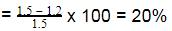∴ % of weak acid in solution = 20%

QUESTION: 6

For the allotropic change represented by the equation C (graphite) → C (diamond), ΔH = 1.9 kJ. If 6 g of diamond and 6 g of graphite are separately burnt to yield CO2, the heat liberated in first case is

Solution:

∆H given in the question is for one mole of C (g). If 6 gm of diamond and graphite are burnt in oxygen then the C (diamond) will first convert to graphite and then it will form CO2. While C (graphite) will directly form CO2. So due to the conversion of diamond into graphite, we will get extra heat. And since we have 6gm (0.5 mol) of diamond, so the heat released will be 0.5×1.9 kJ or 0.95 kJ more than the second case.

QUESTION: 7

If x1, x2 and x3 are enthalpies of H - H, O = O and O - H bonds respectively, and x4 is the enthalpy of vaporisation of water, estimate the standard enthalpy of combustion of hydrogen

Solution:

Combustion of hydrogen:
H2 + ½ O2→H2O (H−O−H)
As water contains two O−H bonds.
So, combustion enthalpy of hydrogen is:
ΔH= Bond energy of reactant − Bond energy of the product − Enthalpy of vaporization.
ΔH=x1+22−2x3−x4

QUESTION: 8

NH3(g) + 3Cl2(g)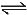NCl3(g) + 3HCl(g) ; -ΔH1

N2(g) + 3H2(g)2NH3(g) ; ΔH2

H2(g) + Cl2(g)2HCl(g) ; ΔH3

The heat of formation of NCl3 (g) in the terms of ΔH1, ΔH2 and ΔH3 is

Solution:

The formation of NCl3 be like
½ N2 + 3/2Cl2 ⇋ NCl3
We can see that for this setup, we need to have eqn (ii) divided by 2, reversing eqn (iii) and multiplying it by 3/2 and then adding all these to equation (i).
So option a is correct.

QUESTION: 9

The enthalpy of neutralisation of HCl and NaOH is -57 kJ mol-1. The heat evolved at constant pressure (in kJ) when 0.5 mole of H2SO4 react with 0.75 mole of NaOH is equal to

Solution:

-57 kJ of heat evolved when 1 mole of NaOH reacted with an acid.
We have 1 mole of H+ and 0.75 moles of OH-. So OH- iis limiting reagent. Or only 0.75 moles of OH- will be used. So for 1 mole of OH-, we have  -57 kJ heat released. Therefore for 0.75 moles, we have ¾× -57 kJ heat released.

QUESTION: 10

Reaction involving gold have been of particular interest to a chemist. Consider the following reactions.

Au(OH)3 + 4 HCl → HAuCl4 + 3H2O,         ΔH = -28 kcal

Au(OH)3 + 4 HBr → HAuBr4 + 3 H2O,       ΔH = -36.8 kcal

In an experiment there was an absorption of 0.44 kcal when one mole of HAuBr4 was mixed with 4 moles of HCl. What is the percentage conversion of HAuBr4 into HAuCl4 ?

Solution:

Au(OH)3 + 4HCl → HAuCl4+ 3H2O…(1) ∆H₁=-28kcal
Au(OH)3 + 4HBr → HAuBr4 + 3H2O ...(2) ∆H₂= -36.8kcal
To convert HAuBr4 to HAuCl4, the net reaction is
HAuBr4 + 4HCl→ HAuCl4 + 4HBr ...∆H=?
For the above reaction:
∆H =∆H₁ - ∆H₂
= -28 - (-36.8) = 8.8 kcal
Thus to convert one mole of HAuBr4 to HAuCl4 we require 8.8 kcal energy but since the energy absorbed is 0.44 kcal.
hence %conversion =[(0.44)/(8.8)] x 100 = 5%
Hence the percentage conversion is 5%.

QUESTION: 11

(i) Cis - 2 - butene → trans - 2 - butene,    ΔH1

(ii) Cis - 2 - butene → 1 - butene,  ΔH2

(iii) Trans - 2 - butene is more stable than cis - 2 - butene.

(iv) Enthalpy of combustion of 1 - butene, ΔH = -649.8 kcal/mol

(v) 9ΔH1 + 5 ΔH2 = 0

(vi) Enthalpy of combustion of trans 2 - butene, ΔH = -647.0 kcal/mol

Q. The value of ΔH1 & ΔH2 in Kcal/mole are

Solution:

Trans-2-butene + 6O2(g) → 4CO2(g) + 4H2O(l)
∆H = -647.0 kcal/mol ---(I)
1 - butene + 6O2(g) → 4CO2(g) + 4H2O(l)
ΔH = -649.8 kcal/mol ---(II)
On (I)-(II)
Trans-2-butene → 1 - butene
ΔH = 2.7 kcal/mol
ΔH = (Hf)1-Butene - (Hf)trans-2-butene
= H2 - H1
H2 - H1 = 2.7 -----(A)
And 9ΔH1 + 5ΔH2 = 0 -----(B)
On solving eqn (A) and (B), we get
H1 = -1.0 and H2 = 1.8

QUESTION: 12

The reaction CH4(g) + Cl2(g) → CH3Cl(g) + HCl(g) has ΔH = -25 kCal.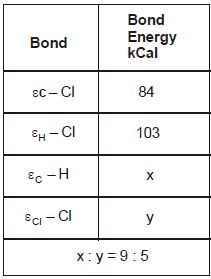From the given data, what is the bond energy of Cl - Cl bond

Solution:

During bond breakage energy is absorbed and during bond formation it is released. From the reaction we can say that 1 C-H bond is broken 1 Cl-Cl bond is broken 1 c-cl bond is formed and 1 h-cl bond is formed. so using the sign conventions the equation becomes
x+y-84-103= -25 (∆H = -25)
5x=9y ..putting x=9/5y we get y = 57.75 kCal

QUESTION: 13

From the following data at 25°C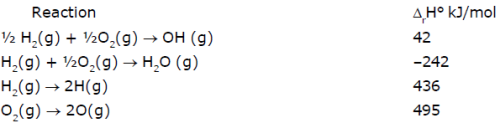Which of the following statement(s) is/are correct :
Statement(a) : ΔrH° for the reaction H2O(g) → 2H(g) + O(g) is 925 kJ/mol
Statement(b) : ΔrH° for the reaction OH(g) → H(g) + O(g) is 502 kJ/mol
Statement(c) : Enthalpy of formation of H(g) is-–218 kJ/mol
Statement(d) : Enthalpy of formation of OH(g) is 42 kJ/mol

Solution: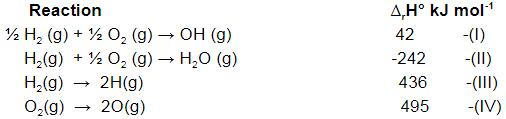Let's check statement (a)
Statement(a) : ΔrH° for the reaction H2O(g) → 2H(g) + O(g) is 925 kJ/mol
For this, we need - (II) + (III) + ½ (IV)
We get, H2O(g) → 2H(g) + O(g) - (-242)+436+½ 495 = 925.5 kJ mol-1
So it is true.
Let's check statement (b)
Statement(b) : ΔrH° for the reaction OH(g) → H(g) + O(g) is 502 kJ/mol
For this we need -(I)+½ (III)+½ (IV)
We get OH(g)   →   H(g)  + O(g)       -(42) + ½ (436) + ½ (495) = 423.5 kJ mol-1
So statement (b) is wrong.
Let's check statement (c)
Statement(c) : Enthalpy of formation of H(g) is -218 kJ/mol
We can see that for enthalpy of formation, we need to divide eqn (III) by 2
So, it would become :-
½ H2(g) → H(g)
436/2 = 218 kJ
So, statement (c) is wrong.
Let's check statement (d)
Statement(d) : Enthalpy of formation of OH(g) is 42 kJ/mol
For that, we have eqn (I) as it is. So, statement (d) is correct.

QUESTION: 14

The standard molar enthalpies for formation of cyclohexane (l) & benzene (l) at 25°C are -156 & + 49 kJ/mol respectively. The standard enthalpy of hydrogenation of cyclohexane (l) at 25° is -119 kJ mol-1. Use these data to estimate the magnitude of the resonance energy of benzene.

Solution:
QUESTION: 15

For hypothetical reaction -

A(g) + B (g) → C (g) + D (g)

Which of the following statements is correct -

Solution:
QUESTION: 16

ΔH for CaCO3(s) → CaO(s) + CO2(g) is 176 kJ mol-1 at 1240 K. The ΔU for the change is equal to :

Solution:

∆H = ∆E + ∆ngRT
176 = ∆E + 1×8.314×1240/1000
∆E = 176 - 10.30 = 165.69 kJ

QUESTION: 17

Select the correct order in the following :

Solution:

107 erg = 1 joule and 4.2 joules = 1 cal
Therefore 1 cal > 1 joule > 1 erg

QUESTION: 18

When enthalpy of reactants is higher than product then reaction will be

Solution:

The enthalpies of these reactions are less than zero, and are therefore exothermic reactions. A system of reactants that absorbs heat from the surroundings in an endothermic reaction has a positive \(ΔH\), because the enthalpy of the products is higher than the enthalpy of the reactants of the system.

QUESTION: 19

In the combustion of 4g. of CH4, 2.5 K cal of heat is liberated. The heat of combustion of CHis -

Solution:

Mol. wt. of methane =16gm
Heat liberated during the combustion of 4gm methane = 2.5kcal
Heat liberated during the combustion of 16gm methane = 2.5/4×16=10kcal
Hence the heat of combustion of methane is 10 kcal.

QUESTION: 20

Ammonium nitrate can decompose with explosion by the following reaction.

NH4NO3 (s) → N2O (g) + 2H2O ;

ΔH = -37.0 KJ/mol

Calculate the heat produced when 2.50g of NH4NO3 decomposes -

Solution:

NH4NO3 (s) → N2O (g) + 2H2O ;     ΔH = -37.0 KJ/mol from the above data, we can show that ΔH is for 1 mole of NH4NO3.
For 2.5 g of NH4NO3, we have 2.5/80 = 0.03125 moles
Therefore, heat released = (-37)×(0.03125) = -1.16 kJ

QUESTION: 21

From the following data, the heat of formation of Ca(OH)2(s) at 18°C is ………..kcal: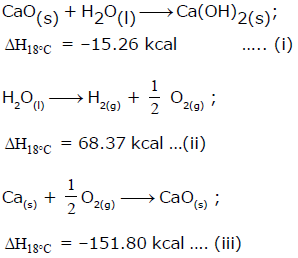Solution:

The correct answer is Option B.

Ca(s) + O2(g) + H2(g) → Ca(OH)2 , ΔHf = ?

Desired equation = eq (iii) + eq(i) - eq (ii)

ΔHf = (−151.80)+(−15.26)−(−68.37)
ΔHf = (-151.80)+(-15.26)-(-68.37)
ΔHf = −235.43KCalmol−1

QUESTION: 22

For the process, melting of ice at 260 K the ΔH is -

Solution:
QUESTION: 23

Enthalpy of formation of compound is -

Solution:

This is not fixed. It depends from compound to compound.
A negative enthalpy of formation indicates that the formation of a compound is exothermic---the amount of energy it takes to break bonds is less than the amount of energy that is released when making the bonds.
A positive enthalpy of formation indicates that the formation of a compound is endothermic---the amount of energy it takes to break bonds is greater than the amount of energy that is released when making the bonds.

QUESTION: 24

The enthalpy of combustion of a substance -

Solution:

The reason behind is that combustion reactions are always exothermic. So the enthalpy of combustion is always less than zero.

QUESTION: 25

HA + OH- → H2O + A- + q1 kJ

H+ + OH- → H2O + q2 kJ

The enthalpy of dissociation of HA is

Solution:

HA + OH⁻ → H₂O + A⁻ + q1 -----(1)
H₂O + q2 → H⁺ + OH⁻ -----(2)
HA + q2 → H⁺ + A⁻  + q1
Hence , bond dissociation energy of HA is (q2 - q1)

QUESTION: 26

The value of ΔHsol. of BaCl2(s) and BaCl2. 2H2O (s) are – a kJ and b kJ respectively. The value of ΔH Hydration of BaCl2 (s) is-

Solution:

BaCl2(s) + aq. ⟶ BaCl2 aq.
∆H = -a kJ ------(i)
BaCl2 2H2O(s) + aq. ⟶ BaCl2 aq. + 2H2O
∆H = b kJ -----(ii)
Enthalpy of hydration means that the molecule is just hydeedratt
The reaction should be
(i) - (ii) or
BaCl2(s) + 2H2O ⟶ BaCl2.2H2O       ∆H = -a - b kJ

QUESTION: 27

A solution of 500 ml of 0.2 M KOH and 500 ml of 0.2 M HCl is mixed and stirred; the rise in temperature is T1. The experiment is repeated using 250 ml of each solution, the temperature raised is T2. Which of the following is true -

Solution:

Let heat evolved in the 1st case is Q1 and that in the 2nd case is Q2. Then Q2 = 1/2Q1
However, Q1 = 1000T1 and Q2 = 500T2
Therefore, 500T2 = ½ 1000T1 i.e. T1 = T2

QUESTION: 28

The net heat change in a chemical reaction is same whether it is brought about in two or more different ways in one or several steps. It is known as -

Solution:

According to Hess law, the thermal effects of a reaction depends upon the initial and final conditions of the reacting substances. It does not depend upon the immediate steps.

QUESTION: 29

According to Hess's Law the thermal effect of a reaction depends on -

Solution:

According to Hess law, the thermal effects of a reaction depends upon the initial and final conditions of the reacting substances. It does not depend upon the immediate steps.

QUESTION: 30

How many kcal of heat is evolved by the complete neutralisation of one mole sulphuric acid with NaOH -

Solution:

For the reaction of 1 mole of H+ and OH-,we have 13.6 kcal energy released. In H2SO4, we have 2 moles of H+. So for its complete neutralisation, we need 2 moles of NaOH.
So in the end, 2 moles of H+ reacts with2 moles of OH- and 13.6 2 = 27.4 kcal energy is released.